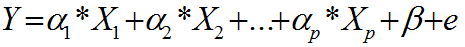# Tag Archives | Multiple Linear regression## Multiple Linear Regression

What is Multiple Linear Regression? Multiple linear regression is a statistical technique to model the relationship between one dependent variable and two or more independent variables by fitting the data set into a linear equation. The difference between simple linear regression and multiple linear regression: Multiple Linear Regression Equation Where: Both dependent and independent variables […]